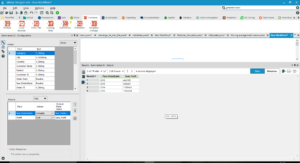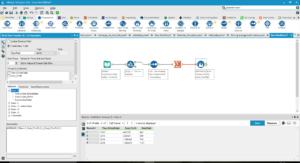## Calculating a Moving Average with Alteryx

by Jeremy Kneebone

To calculate a moving average in Alteryx, follow these steps:

1. Get your data into at least two columns: a date field and the sum of something by date. In this case I have Year and Profit for Dar es Salaam from the Global Superstore data set.
2. Sort your rows so that they are Ascending by the date field.1. Drag a Multi-Row Formula to the canvas.
2. In the configuration of your Multi-Row Formula, set the Num Rows to the number of date parts you want the moving average to calculate over. For example, if you want a two-year moving average and your date field is Year, then set Num Rows to 2.
3. Set Values for Rows that don’t Exist to ‘Set to Values of Closest Valid Row’.
4. Now you need to write your formula. Under Variables, you’ll see drop-downs for Row-2, Row-1, etc. Write the AVERAGE function, then open each of the drop-downs that you need and select the field you want to calculate the moving average of. For example, if you want to calculate the moving average of Profit over the past two years, double-click on Row-1 SUM(Profit), then write a comma, then double-click on Active Row SUM(Profit), then close the brackets.1. Run the workflow, and Alteryx will calculate your moving average.Jeremy Kneebone

Mon 29 Jan 2018

Wed 13 Dec 2017

Mon 11 Dec 2017